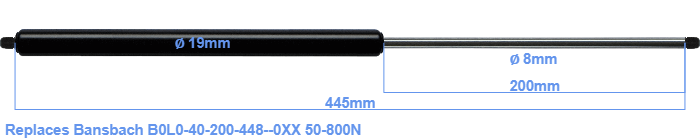# Replacement for Bansbach B0L0-40-200-448–0XX 50-800N

\$34.07

Replacement gas spring for the Bansbach B0L0-40-200-448--0XX 50-800 Newton. Thread: M8. Brand: Hahn.
 Force Choose an option50 Newton60 Newton80 Newton100 Newton120 Newton140 Newton150 Newton160 Newton180 Newton200 Newton220 Newton240 Newton250 Newton260 Newton280 Newton300 Newton320 Newton340 Newton350 Newton360 Newton380 Newton400 Newton420 Newton440 Newton450 Newton460 Newton480 Newton500 Newton520 Newton540 Newton550 Newton560 Newton580 Newton600 Newton620 Newton640 Newton650 Newton660 Newton680 Newton700 Newton720 Newton740 Newton750 Newton760 Newton780 Newton800 NewtonClearThe diameter of the cylinder of this gas spring is 19 mm. The diameter of the rod is 8 millimeter. The stroke (the retracting part) has a length of 200 mm. The total length of this gas strut is 445 mm. This is the distance between the centers of the mounting parts. The length from thread to thread (exlcuding the mounting parts) is 445 millimeter. This replacement gas spring has a force of 50-800 Newton. Attention: this is not an official Bansbach gas spring, but a replacement gas spring. This is a Stabilus Industry Line gas spring. The gas spring can be used without problems as a replacement, because the dimensions and force equal.